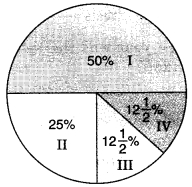• Bar-graph
• Pie-chart
• Histograph
• Line Graph

• Bar-graph
• Pie-chart
• Histograph
• Line Graph

• Linear graph
• Pie-chart
• Histograph
• Bar-graph

• (-2, 0)
• (2, 0)
• (0, -2)
• (2, -2)
• #### 5.If we join (-3,2), (-3,-3) and (-3,4), then we obtain:

• A triangle
• Straight-line without passing through origin
• Straight-line passing through origin
• None of the above
• #### 6.The point (4, 0) lies on which of the following?

• x-axis
• y-axis
• origin
• None of the above
• #### 7.The point (-2,-2) is:

• near to x-axis
• near to y-axis
• near to origin
• Equidistant from x-axis and y-axis
• #### 8.The point (-2, 5) is nearer to:

• x-axis
• y-axis
• origin
• None of the above
• #### 9.The point (-5, 2) is nearer to:

• x-axis
• y-axis
• origin
• None of the above
• #### 10.The point (0, 0) lies at:

• x-axis
• y-axis
• origin
• None of the above
Report Question
Practice More On Introduction to Graphs
access_time